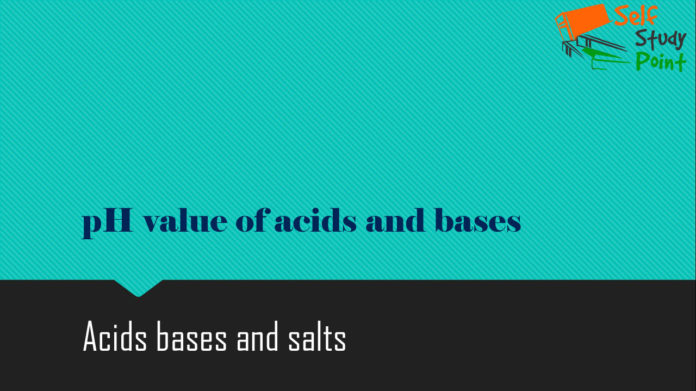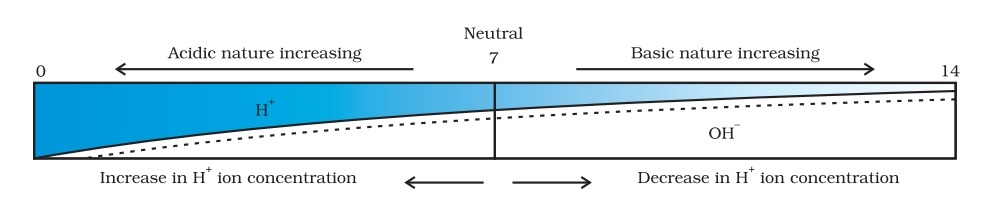If anybody asks you to find out the more strong or weak acid/base. How will you find it out?
The answer is with pH value you can find acidic or alkaline nature of solutions.

## How strong are acid or base solution?

We knew to know the nature of the solution. That means you can find that given solution is acid or base. You can do this with the help of Litmus paper.

But what if somebody asks how strong acidic the solution. If you have two solutions of same acid or base with different concentrations. How are you find which solution is strong or weak?

We can do this by making use of a universal indicator, which is a mixture of several indicators. The universal indicator shows different colors at different concentrations of hydrogen ions in a solution. Which is also called as pH scale.

## pH value:

A scale for measuring hydrogen ion concentration in a solution, called pH scale has been developed. The pH stands for the power of hydrogen.On the pH scale, we can measure pH from 0 (very acidic) to 14 (very alkaline). pH should be thought of simply as a number which indicates the acidic or basic nature of a solution. Higher the hydronium ion concentration, lower is the pH value.
The pH of a neutral solution is 7. Values less than 7 on the pH scale represent an acidic solution. As the pH value increases from 7 to 14, it represents an increase in OH– ion concentration in the solution, that is, increase the strength of alkali.

## pH of Salts:

Salts of a strong acid and a strong base are neutral with a pH value of 7. On the other hand, salts of a strong acid and weak base are acidic with pH value less than 7 and those of a strong base and weak acid are basic in nature, with pH value more than 7.